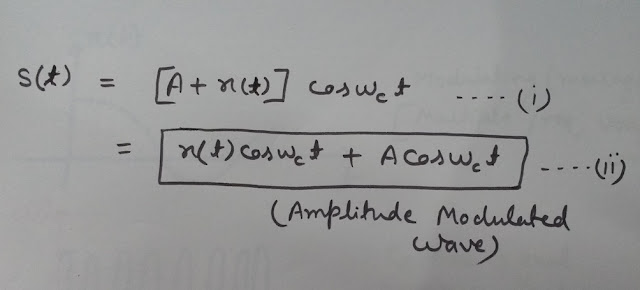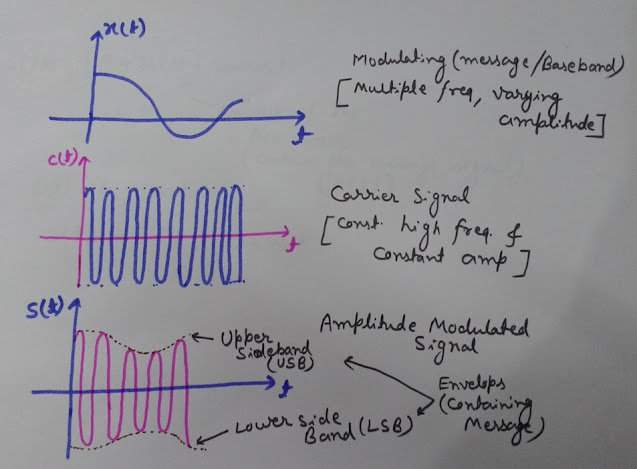# What is Amplitude Modulation (AM)

Definition:-
Amplitude Modulation may be defined as a system, where the maximum amplitude of the carrier wave varies, according to the instantaneous value (amplitude) of the modulating (message or baseband) signal.
Note: [In case of Frequency modulation (FM), the frequency of the carrier wave varies, according to the instantaneous value of the modulating signal, while in Phase Modulation (PM), the phase of the carrier wave varies, according to the instantaneous value of the modulating signal.]

# Amplitude Modulation (AM modulation) Video [HD]

## What Will You Learn Here about Amplitude Modulation?

After reading this post, you will understand:-
#What is Amplitude Modulation (AM)
#Time Domain analysis of the Amplitude Modulation
#Equations of Amplitude Modulation (Time Domain)
#Waveforms of Modulating signal, Carrier signal and Amplitude Modulated wave and
#Properties of the amplitude modulation

# Equations of Amplitude Modulation (Time Domain)

As per the definition of the amplitude modulation we can express it mathematically as-

Let's x(t) is the Modulating signal (also called as Message signal or Baseband signal)
c(t) = Acos(wct)   Carrier wave
Here ‘A’ is the amplitude of the carrier wave and
‘wc’ is the angular frequency of the carrier wave

#AMPLITUDE MODULATION (FREQUENCY DOMAIN ANALYSIS, WAVEFORMS AND EQUATIONS DERIVATION)

Here you can see in the equations, that the carrier wave has a constant amplitude (A) and constant high frequency (wc).
But the Modulating signal (message signal) can have multiple frequencies and variable amplitude.
So now as per the definition of amplitude modulation; let’s now superimpose this modulating signal over the high frequency carrier wave’s amplitude.
See the Image (1) below-Equation of Amplitude Modulation in Time Domain Image (1)

s(t) = [A+x(t)]cos(wct)
=x(t) cos(wct)+ Acos(wct)…(i)
Here in these equations, we have superimposed the amplitude of the carrier wave by the message signal x(t). This superimposition is shown in the equation by putting the modulating signal x(t) with the Amplitude of the Carrier wave.

## Amplitude Modulation Waveform

Now I'm going to show you, some waveforms of modulating signal, Carrier signal and amplitude modulated wave.
See the Image given below to view the Waveforms (Click the Image to enlarge)Amplitude Modulation Waveforms in Time Domain Image (2)

All the waveforms shown here are in Time Domain-
Here are some observations and properties of the waveforms shown in the Image (2) above.

### Modulating Signal x(t) (message signal/baseband signal)

#It has multiple frequencies and
#Variable amplitude

### Carrier Wave c(t)

It has High frequency and
Constant amplitude

### Amplitude Modulated Wave s(t)

As you can see, the waveforms in the Image (2); the amplitude of the high frequency carrier wave gets modulated by the message signal. Here observe, how the variations take place.
Initially the amplitude of the carrier wave was constant but now the amplitude of the carrier wave present in the waveform of the modulated wave s(t), has changed as per the shape of the message signal x(t). You can easily observe that, this shape is same as the shape of the message signal x(t).
The shape that you see here, which is obtained by joining the peak amplitudes of the carrier wave, present in the waveform of amplitude modulated wave s(t), is called as the envelope of the modulated wave. As the shape of this envelope is same as that of the modulating signal x(t), it has the information, that is contained by the modulating signal. This envelope, containing information, can be extracted from the modulated wave at the receiving end by a process called as the Demodulation or detection.
In amplitude modulation, neither the frequency nor the  phase of the carrier wave are changed, only the amplitude of the carrier wave is varied, as per the instantaneous value of the modulating signal. So the information is present in the amplitude variations of the high frequency carrier wave, no information is present in the frequency or phase of the carrier, as both of these are kept constant.
Observe one more thing in the waveform of modulated wave s(t) that it contains two identical shapes similar to the shape of the modulating signal x(t). These are called as the sidebands of the modulated wave and complete information of the message signal is contained by each of the sideband. We can get all the information of the message signal from any of these sidebands. These sidebands are called as the Upper Side Band (USB) and Lower Side Band (LSB).

## Equation of Amplitude Modulated Wave (Envelope Form)

We saw that the equation of AM signal is-
s(t) = [A+x(t)]cos(wct)
Let’s replace [A+x(t)] by E(t)
So we can write the equation of the Amplitude Modulated wave as
s(t) = E(t)cos(wct)
Here E(t) is the envelope of the AM wave, that we have discussed earlier in this post.
Observe here that, envelope contains both, the message signal x(t) and also the amplitude of the carrier wave. So its clear from this equation that the envelope E(t),of the modulated wave contains the message signal x(t), that can be extracted at the receiving end by the process of demodulation.

1.2.